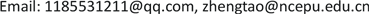1华北电力大学电气与电子工程学院，北京

2国网浙江省电力有限公司台州供电公司，浙江 台州1. 引言

2. 基于传统矩阵算法的配电网故障定位基本原理

2.1. FTU上传信息机制

2.2. 网络描述矩阵D

d i j = { 1 节 点 i 与 j 分 别 为 某 区 段 的 父 、 子 节 点 0 其 他 情 况 (1)

2.3. 故障信息矩阵G

g i i = { − 1   节 点 i 处 FTU 检 测 到 过 电 流 且 方 向 与 全 网 正 方 向 相 反 0 节 点 i 处 FTU 未 检 测 到 过 电 流 1   节 点 i 处 FTU 检 测 到 过 电 流 且 方 向 与 全 网 正 方 向 相 同 (2)

2.4. 故障判断矩阵P

2.5. 故障定位判据

1) 配电网发生单重故障：若 p i i = 1 ，所有的 p i j = 1 ( i ≠ j )，有 p j j = 0 或−1

2) 配电网发生多重故障：若 p i i = 0 ，所有的 p i j = 1 ( i ≠ j )，有 p j j = − 1

1) 配电网发生单重故障：若 p i i = 1 ，所有的 p i j = 0 ( i ≠ j )

3. 基于改进矩阵算法的配电网故障定位基本原理3.1. T接区段对故障定位的影响

3.2. 改进矩阵算法基本原理

1) 通过故障判断矩阵每行的非对角元素，判断哪些节点共同构成T接区段；

2) 通过故障判断矩阵中每行的对角元素，判断T接区段两个子节点FTU上传信息是否相同；

3) 若T接区段两个子节点FTU上传信息不同，则利用等效的思想，在满足T接区段状态不变的前提下，将两个子节点FTU上传信息修正使二者相同，即修正对应的故障判断矩阵对角元素。修正原则为：若两个子节点FTU上传信息不同，只要有一个为“1”，二者都修正为“1”；若没有“1”，只要有一个“−1”，二者都修正为“−1”；

4) 对故障判断矩阵修正后，利用矩阵算法故障定位判据，判断该T接区段是否为故障区段；该T接区段判断结束后，为了避免影响其他区段定位结果，故障判断矩阵修正的对角元素恢复原值。

3.3. 改进矩阵算法故障定位流程

4. 算例分析

D = [ 0 1 0 0 0 0 0 0 0 0 0 0 1 0 1 0 0 0 0 0 0 0 0 1 0 0 0 0 0 0 0 0 0 0 0 0 0 0 0 0 0 0 0 0 0 1 1 0 0 0 0 0 0 0 0 0 0 0 0 0 0 0 0 0 0 0 0 1 1 0 0 0 0 0 0 0 0 0 0 0 0 0 0 0 0 0 0 0 0 1 0 0 0 0 0 0 0 0 0 0 ] (3)

G = [ 1 0 0 0 0 0 0 0 0 0 0 1 0 0 0 0 0 0 0 0 0 0 0 0 0 0 0 0 0 0 0 0 0 0 0 0 0 0 0 0 0 0 0 0 1 0 0 0 0 0 0 0 0 0 0 0 0 0 0 0 0 0 0 0 0 0 − 1 0 0 0 0 0 0 0 0 0 0 − 1 0 0 0 0 0 0 0 0 0 0 − 1 0 0 0 0 0 0 0 0 0 0 − 1 ] (4)

P = D + G = [ 1 1 0 0 0 0 0 0 0 0 0 1 1 0 1 0 0 0 0 0 0 0 0 1 0 0 0 0 0 0 0 0 0 0 0 0 0 0 0 0 0 0 0 0 1 1 1 0 0 0 0 0 0 0 0 0 0 0 0 0 0 0 0 0 0 0 − 1 1 1 0 0 0 0 0 0 0 0 − 1 0 0 0 0 0 0 0 0 0 0 − 1 1 0 0 0 0 0 0 0 0 0 − 1 ] (5)

1) p 11 = 1 ， p 12 = 1 ， p 22 = 1 ，节点1,2构成的双端馈线区段(1)正常；

2) p 22 = 1 ， p 23 = p 25 = 1 ，节点2,3,5构成T接区段(2)；

p 22 = 1 ， p 23 = 1 ， p 33 = 0 ，T接区段(2)故障；

p 22 = 1 ， p 25 = 1 ， p 55 = 1 ，T接区段(2)正常；综上考虑，可得T接区段(2)错判。

3) p 33 = 0 ， p 34 = 1 ， p 44 = 0 ，节点3,4构成的双端馈线区段(3)正常；

4) p 55 = 1 ， p 56 = p 57 = 1 ，节点5,6,7构成T接区段(5)；

p 55 = 1 ， p 56 = 1 ， p 66 = 0 ，T接区段(5)故障；

p 55 = 1 ， p 57 = 1 ， p 77 = − 1 ，T接区段(5)故障；综上考虑，可得T接区段(5)故障。

1) p 22 = 1 ， p 23 = p 25 = 1 ，节点2,3,5构成T接区段(2)；

p 33 = 0 ， p 55 = 1 ，两个子节点3,5处FTU上传信息不同；

p 33 = p 55 = 1 ，两个子节点3,5处FTU上传信息修正；

p 22 = 1 ， p 23 = 1 ， p 33 = 1 ，T接区段(2)正常；

p 22 = 1 ， p 25 = 1 ， p 55 = 1 ，T接区段(2)正常；综上考虑，可得T接区段(2)正常。

2) p 55 = 1 ， p 56 = p 57 = 1 ，节点5,6,7构成T接区段(5)；

p 66 = 0 ， p 77 = − 1 ，两个子节点6,7处FTU上传信息不同；

p 66 = p 55 = − 1 ，两个子节点6,7处FTU上传信息修正；

p 55 = 1 ， p 56 = 1 ， p 66 = − 1 ，T接区段(5)故障；

p 55 = 1 ， p 57 = 1 ， p 77 = − 1 ，T接区段(5)故障；综上考虑，可得T接区段(5)故障。

Fault location simulation resul

1区段(8)[1 1 0 0 1 0 1 −1 1 −1]p 99 = 1 ， p 9 , 10 = 1 ， p 10 , 10 = − 1区段(8)
2区段(6)[1 1 0 0 1 1 −1 −1 −1 −1]p 66 = 1区段(6)
3区段(5)[1 1 0 0 1 0 −1 −1 −1 −1]p 55 = 1 ， p 56 = p 57 = 1 ，区段(5)
p 66 ≠ p 77 ， p 66 = p 77 = − 1 ，
p 55 = 1 ， p 56 = 1 ， p 66 = − 1
p 55 = 1 ，
4区段(7)[1 1 0 0 1 0 1 −1 −1 −1]区段(7)
5区段(1) (8)[1 −1 0 0 −1 0 −1 −1 1 −1]区段(1)

5. 结语